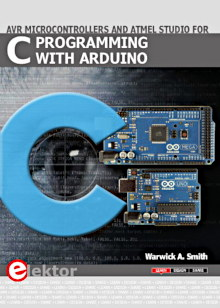Starting Electronics needs your help! Please make a donation to help cover our hosting and other costs. Click the donate button to send a donation of any amount.# Tutorial 20: VHDL Case Statement LED Display Sequencer

Created on: 18 March 2013

The VHDL case statement is used to sequence various patterns on eight LEDs. This is the final tutorial in this VHDL CPLD course.

This video shows the VHDL code in operation on a CPLD.

Can't see the video? View on YouTube →

## VHDL Case Statement Code

The code listing for the VHDL case statement LED display is shown below.

```library IEEE;
use IEEE.STD_LOGIC_1164.ALL;
use IEEE.STD_LOGIC_UNSIGNED.ALL;

entity case_display_top is
Generic ( CLK_BIT : INTEGER := 4);
Port ( CLK : in  STD_LOGIC;
LED : out  STD_LOGIC_VECTOR (7 downto 0));
end case_display_top;

architecture Behavioral of case_display_top is
signal clk_div : STD_LOGIC_VECTOR (CLK_BIT downto 0);
signal count   : STD_LOGIC_VECTOR (4 downto 0) := "00000";
begin

-- clock divider
process (CLK)
begin
if (CLK'Event and CLK = '1') then
clk_div <= clk_div + '1';
end if;
end process;

-- counter
process (clk_div(CLK_BIT))
begin
if (clk_div(CLK_BIT)'Event and clk_div(CLK_BIT) = '1') then
count <= count + '1';
end if;
end process;

-- LED display
process (count)
begin
-- display different LED pattern for each count value
case count is
when "00000" => LED <= not "10000001";
when "00001" => LED <= not "01000010";
when "00010" => LED <= not "00100100";
when "00011" => LED <= not "00011000";
when "00100" => LED <= not "00100100";
when "00101" => LED <= not "01000010";
when "00110" => LED <= not "10000001";
when "00111" => LED <= not "11000011";
when "01000" => LED <= not "11100111";
when "01001" => LED <= not "11111111";
when "01010" => LED <= not "11100111";
when "01011" => LED <= not "11000011";
when "01100" => LED <= not "10000001";
when "01101" => LED <= not "00000000";
when "01110" => LED <= not "10000000";
when "01111" => LED <= not "01000000";
when "10000" => LED <= not "01100000";
when "10001" => LED <= not "01100000";
when "10010" => LED <= not "01110000";
when "10011" => LED <= not "01111000";
when "10100" => LED <= not "01111100";
when "10101" => LED <= not "01111110";
-- skip some numbers in count sequence
when "10111" => LED <= not "10101010";
when "11000" => LED <= not "01010101";
when "11001" => LED <= not "10101010";
when "11010" => LED <= not "01010101";
when others => LED <= not "00000000";
end case;
end process;

end Behavioral;
```

A VHDL case statement is sequential, so must be put inside a process.

In the above code, the case statement checks to see if count has a value of "00000", "00001", "00011", etc. If there is a match, then the statement to the right of => will be executed.

The count register counts from 00000b to 11111b and then wraps around to 00000b again. Every count number that matches in the case statement results in a pattern being written to the LEDs.

If there is no matching number for count in the case statement, then the default pattern is displayed which is all the LEDs off as shown in this line of code:

```when others => LED <= not "00000000";
```

Every case statement in VHDL must have a default value using the VHDL others keyword.

Books that may interest you: